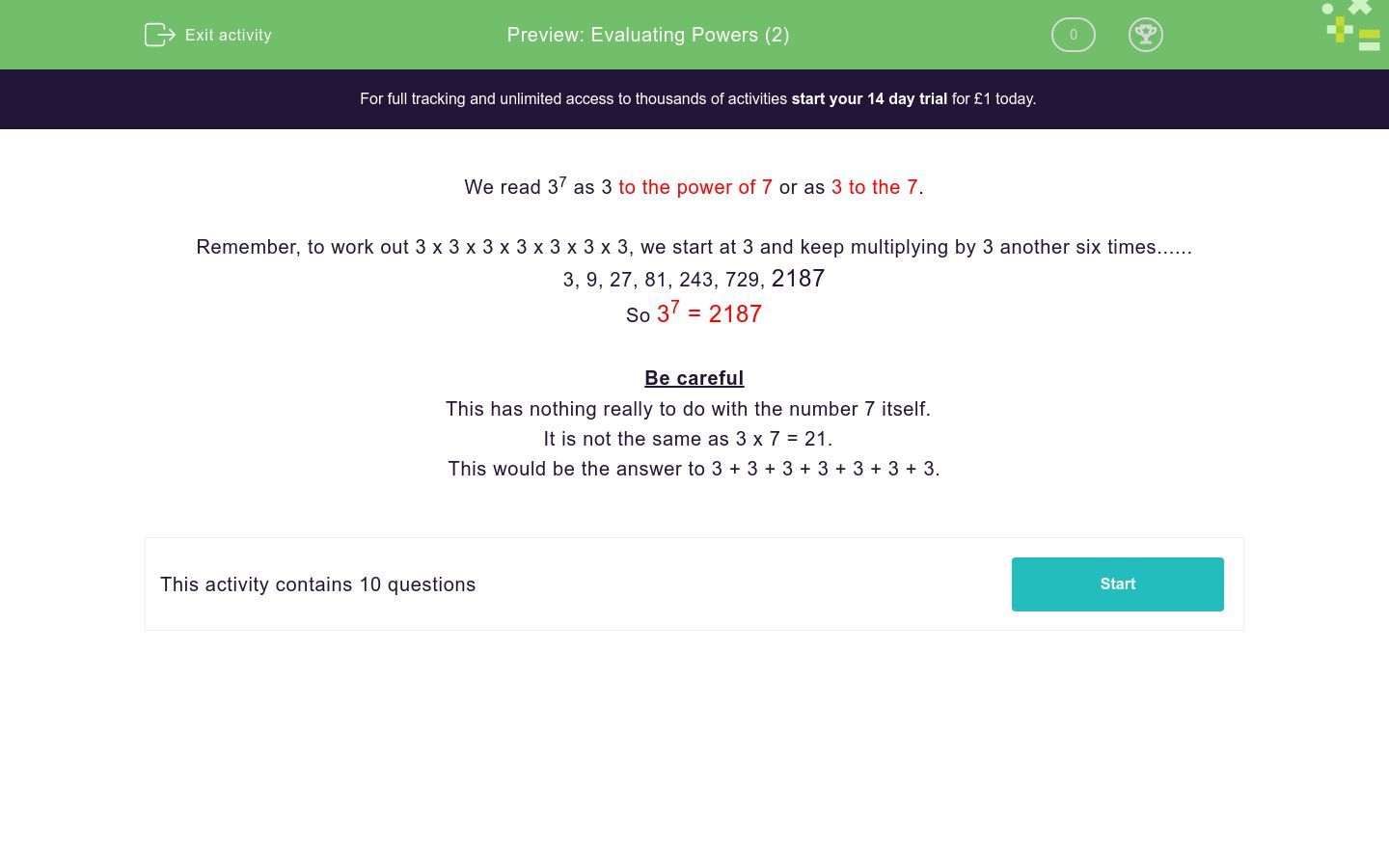# Evaluating Powers (2)

In this worksheet, students evaluate powers.Key stage:  KS 3

Curriculum topic:   Number

Curriculum subtopic:   Understand Integer Powers/Real Roots

Difficulty level:### QUESTION 1 of 10

We read 37 as 3 to the power of 7 or as 3 to the 7.

Remember, to work out 3 x 3 x 3 x 3 x 3 x 3 x 3, we start at 3 and keep multiplying by 3 another six times......

3, 9, 27, 81, 243, 729, 2187

So 37 = 2187

Be careful

This has nothing really to do with the number 7 itself.

It is not the same as 3 x 7 = 21.

This would be the answer to 3 + 3 + 3 + 3 + 3 + 3 + 3.

Work out the value of:

27

128

64

27

14

Work out the value of:

107

70

17

1000000

10000000

Work out the value of:

1010

100

20

10000000000

10000

Work out the value of:

1254

254

24

1

1254

Work out the value of:

02000

2000

1

0

20000

Work out the value of:

43

7

12

43

64

Work out the value of:

44

316

8

16

256

Work out the value of:

54

20

625

125

1000

Work out the value of:

73

147

24

343

21

Work out the value of:

113

1331

121

113

33

• Question 1

Work out the value of:

27

128
• Question 2

Work out the value of:

107

10000000
• Question 3

Work out the value of:

1010

10000000000
• Question 4

Work out the value of:

1254

1
• Question 5

Work out the value of:

02000

0
• Question 6

Work out the value of:

43

64
• Question 7

Work out the value of:

44

256
• Question 8

Work out the value of:

54

625
• Question 9

Work out the value of:

73

343
• Question 10

Work out the value of:

113

1331
---- OR ----

Sign up for a £1 trial so you can track and measure your child's progress on this activity.

### What is EdPlace?

We're your National Curriculum aligned online education content provider helping each child succeed in English, maths and science from year 1 to GCSE. With an EdPlace account you’ll be able to track and measure progress, helping each child achieve their best. We build confidence and attainment by personalising each child’s learning at a level that suits them.

Get started